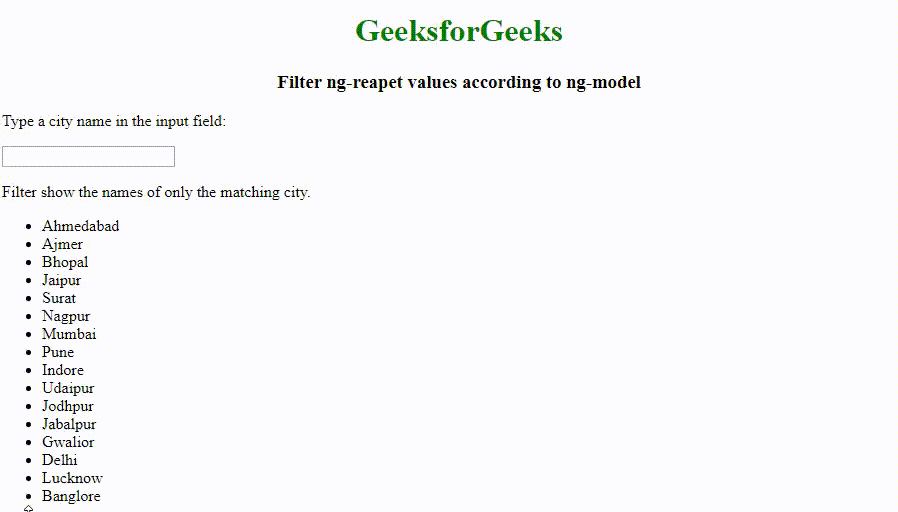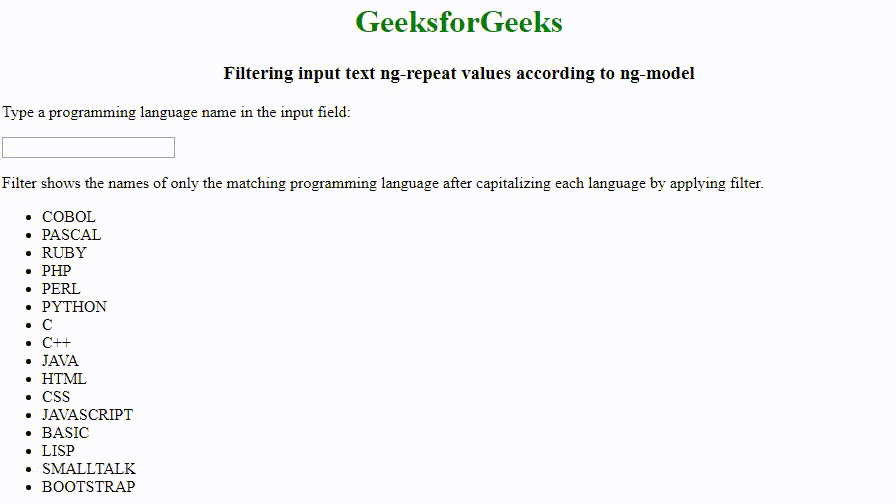# How to filter ng-repeat values according to ng-model using AngularJS ?

The ng-repeat values can be filtered according to the ng-model in AngularJS by using the value of the input field as an expression in a filter. We can set the ng-model directive on an input field to filter ng-repeat values.

Below examples illustrates the approach:
Example 1: Filtering input text ng-repeat values according to ng-model. This filter will show the names of only the matching city

 ` ` `<``html``> ` ` `  `<``head``> ` `    ``<``script` `src``= ` `"https://ajax.googleapis.com/ajax/libs/angularjs/1.6.9/angular.min.js"``> ` `    `` ` ` ` ` `  `<``body``> ` `    ``<``center``> ` `        ``<``h1` `style``=``"color:green;"``>GeeksforGeeks ` `        ``<``h3``>Filter ng-reapet values according to ng-model ` `    `` ` `    ``<``div` `ng-app``=``"app1"` `ng-controller``=``"controller1"``> ` ` `  `        ``<``p``>Type a city name in the input field: ` ` `  `        ``<``p``> ` `            ``<``input` `type``=``"text"` `ng-model``=``"testfilter"``> ` `        `` ` `        ``<``p``>Filter show the names of only the matching city. ` ` `  `        ``<``ul``> ` `            ``<``li` `ng-repeat``=``"x in citynames | filter:testfilter"``> ` `                ``{{ x }} ` `            `` ` `        `` ` ` `  `    `` ` ` `  `    ``<``script``> ` `        ``angular.module('app1', []).controller('controller1', function(\$scope) { ` `            ``\$scope.citynames = [ ` `                ``'Ahmedabad', ` `                ``'Ajmer', ` `                ``'Bhopal', ` `                ``'Jaipur', ` `                ``'Surat', ` `                ``'Nagpur', ` `                ``'Mumbai', ` `                ``'Pune', ` `                ``'Indore', ` `                ``'Udaipur', ` `                ``'Jodhpur', ` `                ``'Jabalpur', ` `                ``'Gwalior', ` `                ``'Delhi', ` `                ``'Lucknow', ` `                ``'Banglore' ` `            ``]; ` `        ``}); ` `    `` ` ` `  ` ` ` `  ` `

Output:Example 2: Filtering input text ng-repeat values according to ng-model. This filter will shows the names of only the matching programming language after capitalizing each language by applying filter.

 ` ` `<``html``> ` `<``head``> ` `    ``<``script` `src``= ` `"https://ajax.googleapis.com/ajax/libs/angularjs/1.6.9/angular.min.js"``> ` `    `` ` ` ` ` `  `<``body``> ` `    ``<``center``> ` `        ``<``h1` `style``=``"color:green;"``>GeeksforGeeks ` `        ``<``h3``>Filtering input text ng-repeat values according to ng-model ` `    `` ` `    ``<``div` `ng-app``=``"app1"` `ng-controller``=``"controller1"``> ` ` `  `        ``<``p``>Type a programming language name in the input field: ` ` `  `        ``<``p``> ` `            ``<``input` `type``=``"text"` `ng-model``=``"testfilter"``> ` `        `` ` `        ``<``p``> ` `          ``Filter shows the names of only the matching programming language ` `          ``after capitalizing each language by applying filter. ` `        `` ` ` `  `        ``<``ul``> ` `            ``<``li` `ng-repeat``=``"x in programminglanguagenames| filter:testfilter"``> ` `                ``{{ x |myfilter}} ` `            `` ` `        `` ` ` `  `    `` ` ` `  `    ``<``script``> ` `        ``var app = angular.module('app1', []); ` `        ``app.filter('myfilter', function() { ` `            ``return function(x) { ` `                ``var i, c, txt = ""; ` `                ``for (i = 0; i < ``x.length``; i++) { ` `                    ``c` `= ``x``[i]; ` ` `  `                    ``c` `= c.toUpperCase(); ` ` `  `                    ``txt += c; ` `                ``} ` `                ``return txt; ` `            ``}; ` `        ``}); ` `        ``app.controller('controller1', function(\$scope) { ` `            ``\$scope.programminglanguagenames = [ ` `                ``'cobol', ` `                ``'pascal', ` `                ``'ruby', ` `                ``'php', ` `                ``'perl', ` `                ``'python', ` `                ``'c', ` `                ``'c++', ` `                ``'java', ` `                ``'html', ` `                ``'css', ` `                ``'javascript', ` `                ``'basic', ` `                ``'lisp', ` `                ``'smalltalk', ` `                ``'bootstrap' ` `            ``]; ` `        ``}); ` `    `` ` ` `  ` ` ` `  ` `

Output:My Personal Notes arrow_drop_upCheck out this Author's contributed articles.

If you like GeeksforGeeks and would like to contribute, you can also write an article using contribute.geeksforgeeks.org or mail your article to contribute@geeksforgeeks.org. See your article appearing on the GeeksforGeeks main page and help other Geeks.

Please Improve this article if you find anything incorrect by clicking on the "Improve Article" button below.